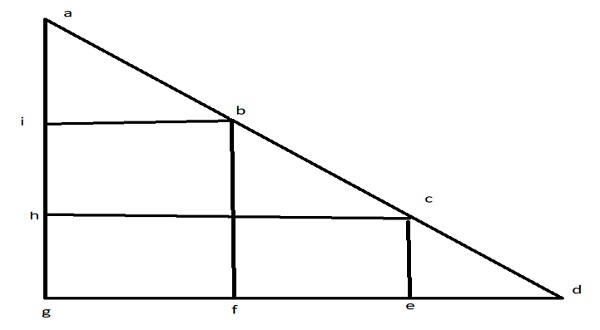# Maximum number of 2×2 squares that can be fit inside a right isosceles triangle in C

We are given a right isosceles triangle. Isosceles triangle is the one which has two sides of equal length. Right triangle is the one which has height(ag in fig.) and base (dg in fig.) perpendicular to each other. The goal is to find the maximum number of squares that can fit into this right isosceles triangle of side 2 sq units. The sides base or height (both equal) are taken as input. No. of squares is output.

Refer to the below figure to understand the problemThe given triangle of height ag and base gd has 3 squares of side 2 each. From the corner ends ‘a’ and ‘d’, triangles aib and cde would never contribute to any square. So first we will always need extra 2 units of length for these triangles. No we have to divide remaining base gd( or height ag) by 2 to count no. of squares. Same will be the case with height ( ag ).

while(base > 2 )
squares+=(base-2)/2
base = base-2.
Use formula of Ap = b*(b+1)/2….where new b=b-2

Let’s understand with examples.

Input − Base: 12

Output − Count of squares : 15

Explanation

base 12>2, squares 10/2=5, new base 12-2=10
base 10>2, squares 8/2=4, new base 10-2=8
base 8>2, squares 6/2=3, new base 8-2=6
base 6>2, squares 4/2=2, new base 6-2=4
base 4>2, squares 2/2=1, new base 4-2=2
base 2>2 X Total squares=5+4+3+2+1=15

Input − .5

Output − Count of squares − 1

Explanation

base 5>2, squares 3/2=1, new base 5-2=3
base 3>2, squares 1/2=0, new base 3-2=1
base 1>2 X Total squares=1

## Approach used in the below program is as follows

• The integer variable base is used to store the Base of triangle..

• Function numofSquares(int b) is used to count the number of squares that triangle with base b can accommodate.

• At first b=b-2 −extra space from corner ends

• According to formula, b=floor(b/2), this new b can have b*(b+1)/2 squares of side 2.

• Return the calculation as the number of squares.

## Example

Live Demo

#include <stdio.h>
#include <math.h>
int numofSquares(int b){
// removing the extra part we would
// always need
b = (b - 2);
// Since each square has base of
// length of 2
b = floor(b / 2);
return b * (b + 1)/2;
}
int main(){
int base = 8;
printf("Maximum no. of square that can be accommodated : %d",numofSquares(base));
return 0;
}

## Output

If we run the above code it will generate the following output −

Maximum no. of square that can be accommodated : 6

Updated on: 17-Aug-2020

483 Views